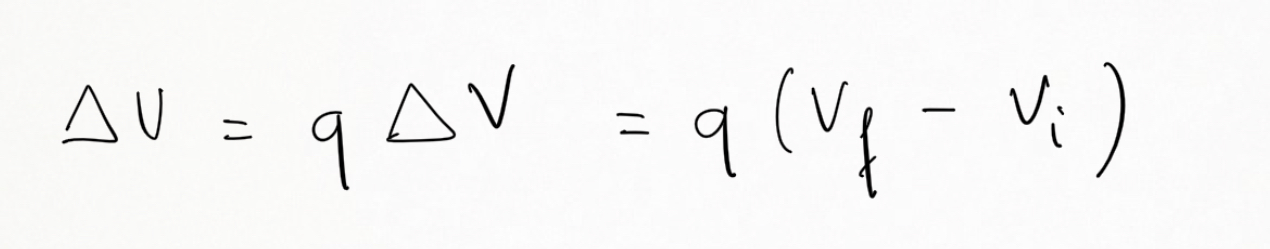Cyber Sale Extended 3 Days: ALL MCAT Products & Admissions Services

MCAT Content / Electrostatics / Electrostatic Energy Electric Potential At A Point In Space

### Electrostatic energy, electric potential at a point in space

Topic: Electrostatics

The electric potential is the amount of electric potential energy per unit charge when a positive test charge is brought in from infinity.

The work done by the electric force, a conservative force, to bring a test charge from infinity to a point close to the source of the electric field is stored as Electrostatic energy (U).

Electric potential (V) is the electrostatic energy at that point of a test charge of (+1C).The electrostatic energy depends on the position and the value of the test charge. Whereas the electric potential energy only depends on the position in the electric field.

If the particle moves through a potential difference ∆􏰌V, the change in the electric potential energyisKey Points

• The electric potential is the amount of electric potential energy per unit charge when a positive test charge is brought in from infinity.

• The electrostatic energy depends on the position and value of the test charge. Electric potential energy only depends on the position in the electric field.

Key Terms

test charge: is a charge with a magnitude so small that placing it at a point has a negligible effect on the field around the point.

conservative force: is a force with the property that the work done in moving a particle between two points is independent of the path it takes.

Billing Information